Ellipses as sections of cylinders: Dandelin Spheres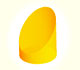We study different cylinders cut by an oblique plane. The section that we get is an ellipse.

We suspect that that the intersection of a plane and a cylinder (not parallel to its axis) is an ellipse.In this page we are going to prove that result using one idea due to Germinal Pierre Dandelin (1794-1847). Dandelin was a Belgian mathematician and military engineer.

We know a lot of things about ellipses. An ellipse is commonly defined as the locus of points P such that the sum of the distances from P to two fixed points F1, F2 (called foci) are constant. We are going to use this definition later.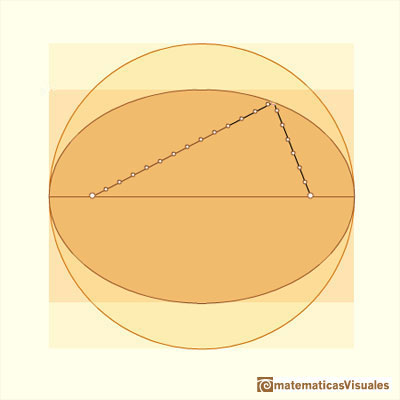Every ellipse has two foci and if we add the distance between a point on the ellipse and these two foci we get a constant.Transforming a circle we can get an ellipse (as Archimedes did to calculate its area). From the equation of a circle we can deduce the equation of an ellipse.

We know how to calculate the area of the ellipse:In his book 'On Conoids and Spheroids', Archimedes calculated the area of an ellipse. We can see an intuitive approach to Archimedes' ideas.

Even we can build mechanical devices to draw ellipses: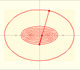An Ellipsograph is a mechanical device used for drawing ellipses.

Dandelin's idea is to consider two spheres inscribed in the cylinder and tangent to the plane that intersect the cylinder. Each of these spheres is tangent to the cylinder in a circle. These circles are parallel and the distance between those circles along any generating line of the cylinder is constant.

These spheres are called Dandelin's spheres.

We shall prove that the points of tangency are the foci of the ellipse.

Playing with the interactive application we can change the distance between the spheres, move the point on the curve and rotate the cylinder.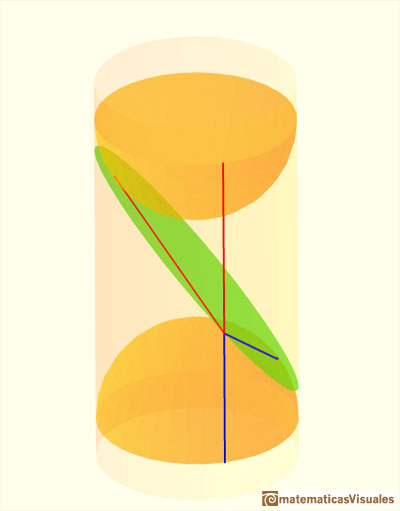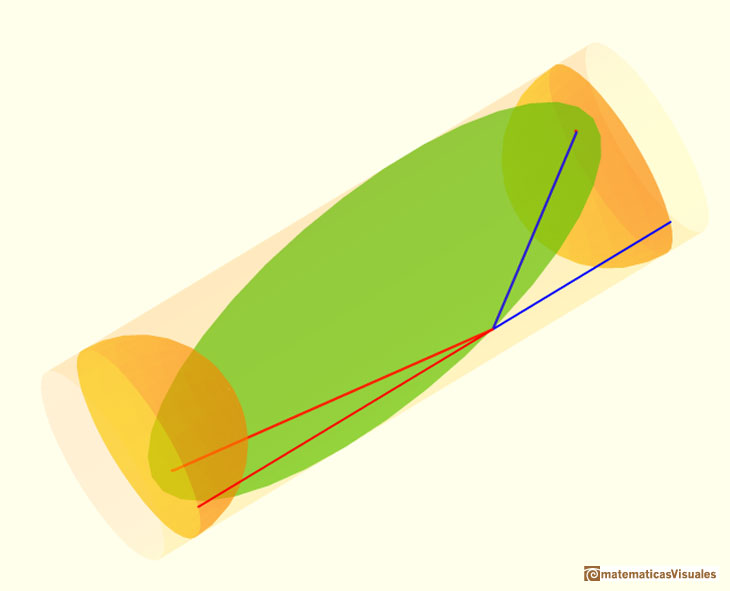We are going to follow Hilbert and Cohn-Vossen's book 'Geometry and the Imagination':

"A circular cylinder intersects every plane at right angles to its axis in a circle. A plane not at right angles to the axis nor parallel to it intersects the cylinder in a curve that looks like an ellipse. We shall prove this curve really is an ellipse. To this end, we take a sphere that just fits into the cylinder, and move it within the cylinder until it touches the intersecting plane (Fig. 9)."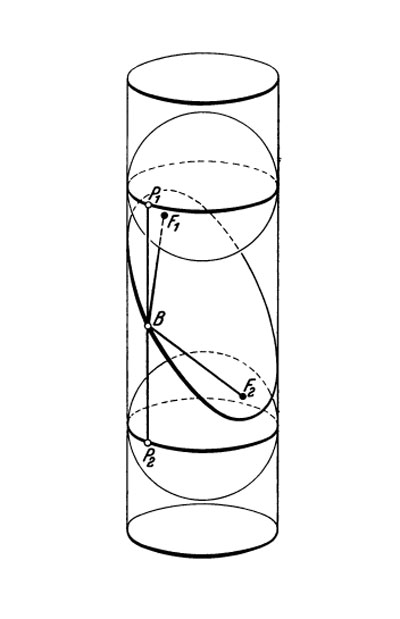Hilbert and Cohn-Vossen. Geometry and the Imagination. Chelsea Publishing Company. pag.7.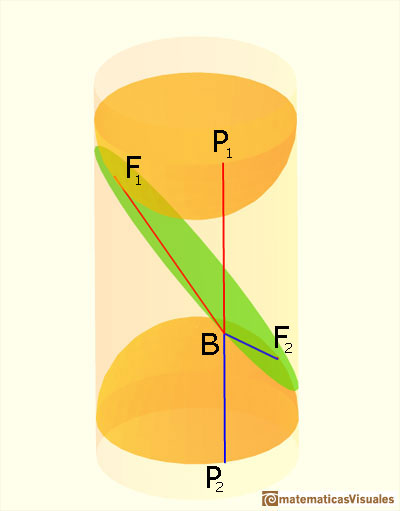"We then take another such sphere and do the same thing with it ont the other side of the plane. The spheres touch the cylinder in two circles and touch the intersecting plane at two points, F1 and F2. Let B be any point on the curve of intersection of the plane with the cylinder. Consider the straight line through B lying on the cylinder (i.e. parallel to the axis). It meets the circle of contact of the spheres at two points P1 and P2. BF1 and BP1 are tangents to a fixed sphere through a fixed point B, and all such tangents must be equal, because of the rotational symmetry of the sphere. Thus BF1=BP1; and similarly BF2=BP2. It follows that

But by the rotational symmetry of our figure, the distance P1P2 is independent of the point B on the curve. Therefore BF1+BF2 is constant for all points B of the section; i.e. the curve is an ellipse with foci at F1 and F2."

"The fact that we have just proved can also be formulated in terms of the theory of projections as follows: The shadow that a circle throws onto an oblique plane is an ellipse if the light rays are perpendicular to the plane of the circle." (Hilbert and Cohn-Vossen. Geometry and the Imagination)

A particular case: the circle (the two foci are the same point that we call the certer of the circle).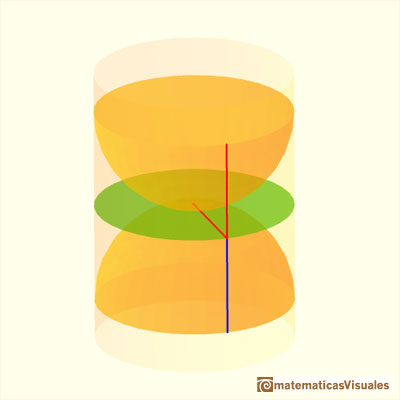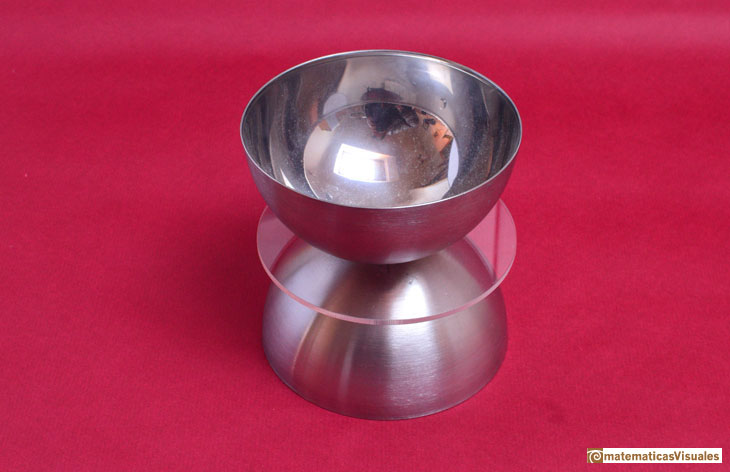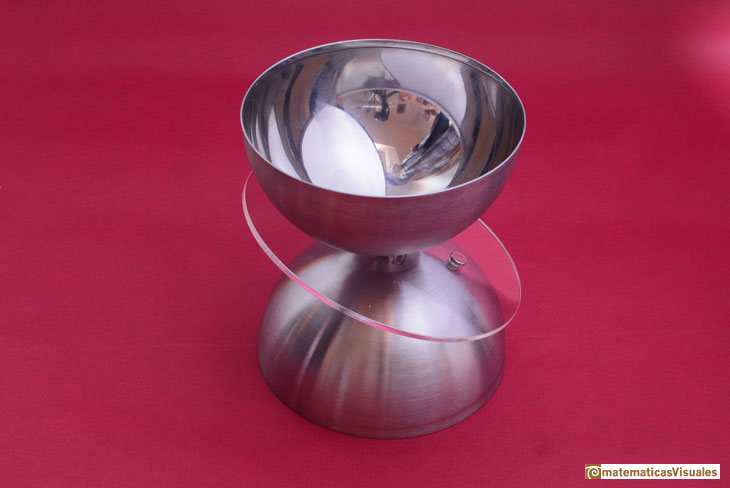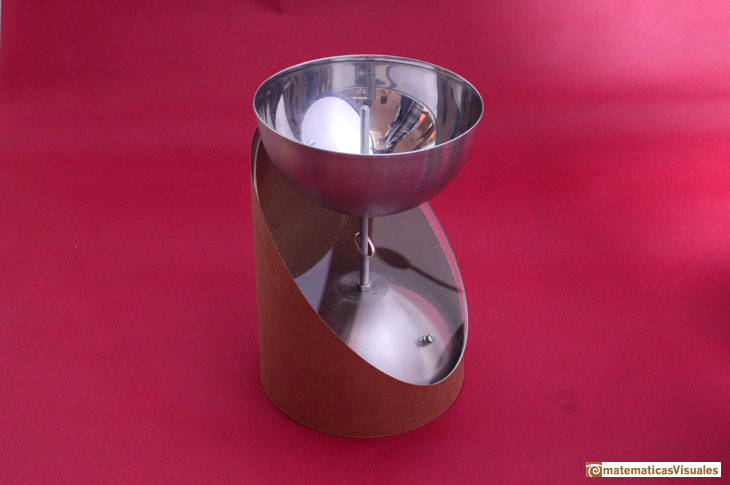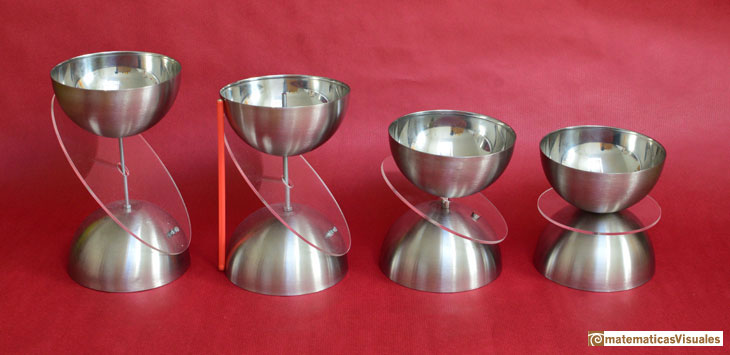More general, the intersection of a plane and a cone is a conic section (ellipse, hyperbola, parabola).Durer was the first who published in german a method to draw ellipses as cone sections.

REFERENCES

Hilbert and Cohn-Vossen. Geometry and the Imagination. Chelsea Publishing Company. pag.7.
Dan Pedoe, Geometry and the Visual Arts. Dover Publications.
C. Stanley Ogilvy, Excursions in Geometry. Oxford University Press.We study different cylinders cut by an oblique plane. The section that we get is an ellipse.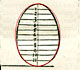Durer made a mistake when he explanined how to draw ellipses. We can prove, using only basic properties, that the ellipse has not an egg shape .In his book 'On Conoids and Spheroids', Archimedes calculated the area of an ellipse. We can see an intuitive approach to Archimedes' ideas.In his book 'On Conoids and Spheroids', Archimedes calculated the area of an ellipse. It si a good example of a rigorous proof using a double reductio ad absurdum.Transforming a circle we can get an ellipse (as Archimedes did to calculate its area). From the equation of a circle we can deduce the equation of an ellipse.An Ellipsograph is a mechanical device used for drawing ellipses.If a straight-line segment is moved in such a way that its extremities travel on two mutually perpendicular straight lines then the midpoint traces out a circle; every other point of the line traces out an ellipse.Plane developments of cones cut by an oblique plane. The section is an ellipse.Plane developments of cones and conical frustum. How to calculate the lateral surface area.We study different cylinders and we can see how they develop into a plane. Then we explain how to calculate the lateral surface area.